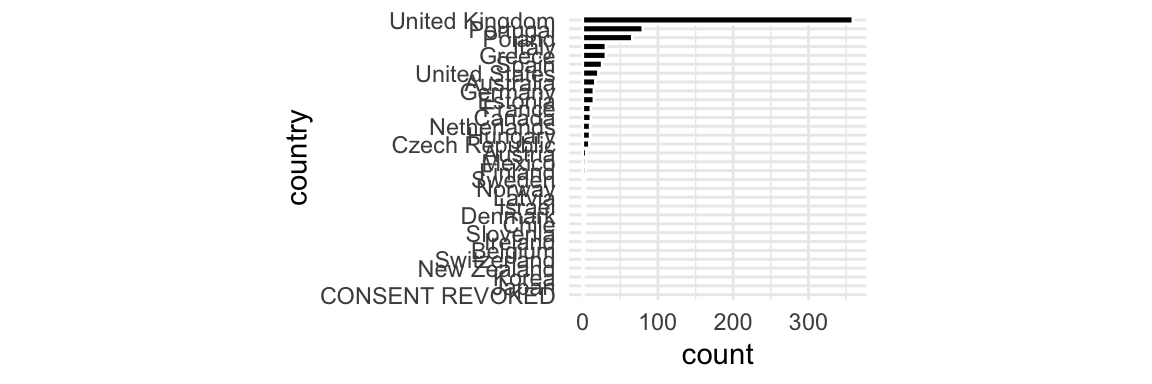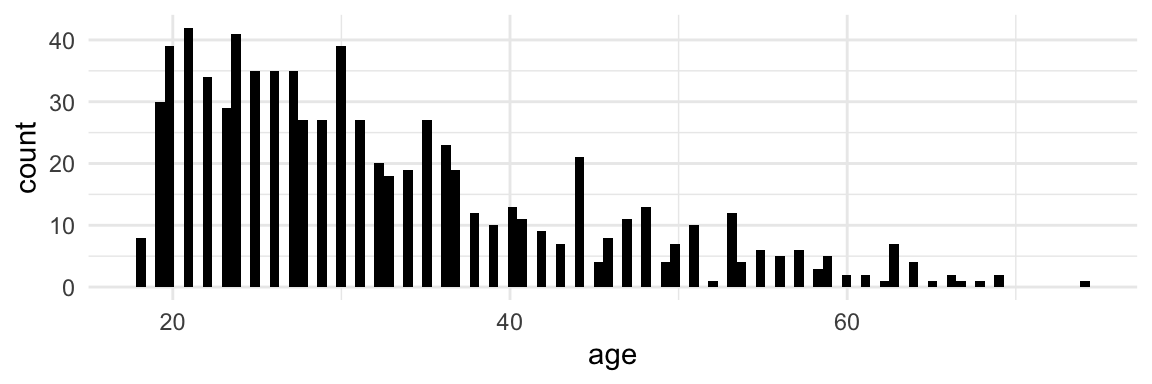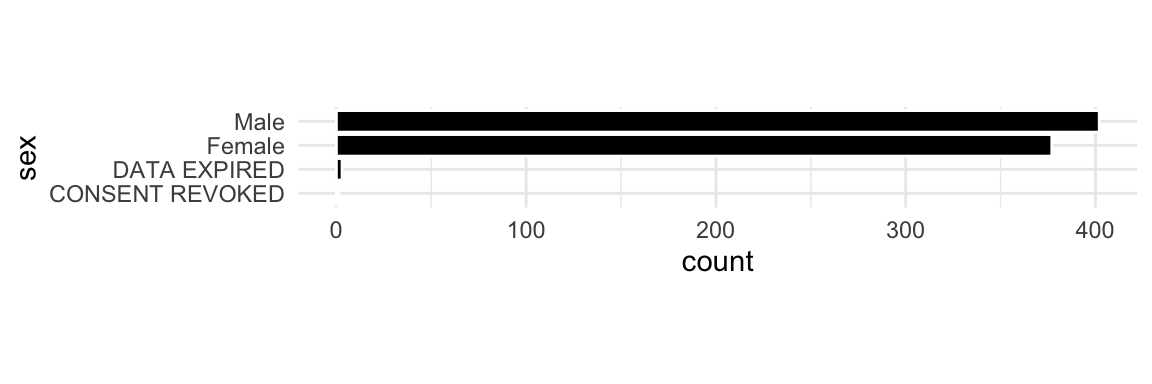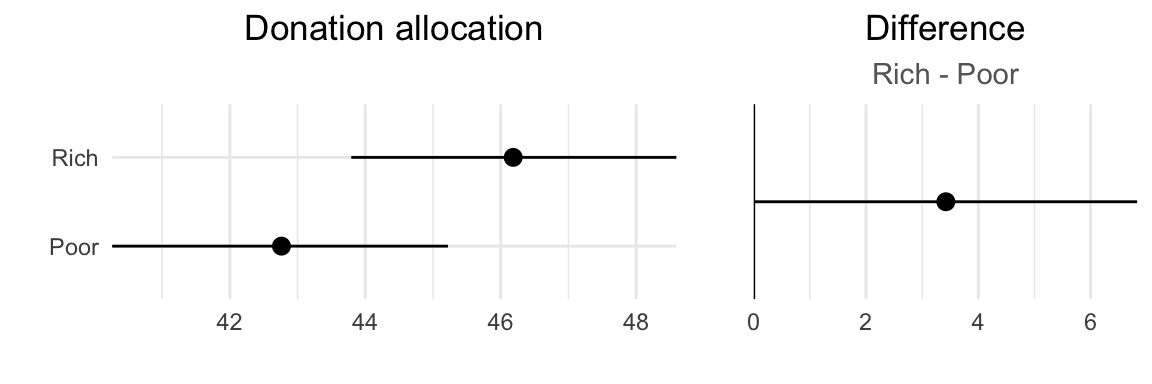This analysis estimates the effect of an information-rich design (as opposed to a statistical chart, which we call information-poor design) on donations and affect.

The following factors and measures were changed in respect to experiment 1:

• We dropped the scenario as a dependent variable and thus use now a purely between-subject design. This is because we didn’t find evidence that the within-subject effect size (looking at differences between the two scenarios) led to a higher statistical power.
• We added the independent variable ‘’identity’’, which controls if the geographical regions in the scenarios are anonymized or named. The free-text comments from the first experiment suggested that pre-existing opinions for or against the mentioned regions played a role in how they allocated funds in experiment 1. We expect that anonymization will reduce the effect of prior attitude.
• In the fund allocation question, we use a different alternative cause to donate to. We observed in the first experiment that many participants attempted to allocate money ‘fairly’ which led to many naive (50/50) allocations. In a formative experiment, we tested six alternative causes to identify which one (when in competition with the cause to help migrants) led to the lowest number of naive allocations.
• We added two affect questions (arousal/valence) at the end of the experiment so that if we don’t find an effect of donation allocation, we can at least assess whether the information-rich visualization has an emotional impact compared to the information-poor visualization.
``````rm(list = ls())

library(NSM3)

library(tidyverse)
# library(scales)
library(cowplot)
library(bootES)

source("helpers.R")
source("CI-helpers.R")
source("histogram.R")

# Completion code used to determine valid submissions
completion_code = "6B23E247"

# Participant IDs used for preview and testing (those will be discarded)
exclude_participant_ids = c("5d8cca80897af7001a72156a", "test")

# set the path to the data you want to use
datapath = "data/"``````

# 1 Data

We read and combine four data files:

• prolific_export.csv is created by Prolific and contains the job status, the completion code entered and basic demographics for all participants who accepted the job.
• results.csv is created by our web server and contains the responses from all participants who completed the job.
• excluded.csv is created by our web server and lists all participants who either failed the attention check or attempted to reload the page. It does not list participants who dropped out for other reasons.
• all_who_agreed.csv is created by our web server and lists all participants who agreed to the consent form, no matter what they did next. We will only consider those participants in our analysis of attrition rates, because many participants who drop before may experience irrelevant issues such as browser compatibility problems.
``````# Load the four data files and only keep the useful columns
dplyr::select(participant_id,
entered_code,
time_taken,
age,
country = `Current Country of Residence`,
sex = Sex)

dplyr::select(participant_id,
donation,
valence,
arousal
) %>%
mutate(donation = 100 - donation) # Donation for cause B

dplyr::select(participant_id,
failed_attention_check,
mutate(participant_id = as.character(participant_id)) # necessary if all are NAs

dplyr::select(participant_id,
condition,
design,
identity)

# Join the four datasets and clean up a bit
data_all = agreed %>%
left_join(excluded, by = "participant_id") %>%
left_join(prolific_export, by = "participant_id") %>%
full_join(results, by = "participant_id") %>%
subset(!participant_id %in% exclude_participant_ids) %>%
mutate(failed_attention_check = coalesce(failed_attention_check, 0), # replace NAs with zeros
mutate(
identity = case_when(
identity == "NonAnonymous" ~ "Named",
identity == "Anonymous" ~ identity
),
design = case_when(
design == "Anthropographic" ~ "Rich",
design == "NonAnthropographic" ~ "Poor"
),
condition = paste(identity, design, sep = "_")
)

# Clean up possibly serious anomalies in the data and issue warnings if necessary
data_all_original = data_all
data_all = data_all %>%
drop_na(participant_id, condition) %>%
distinct(participant_id, .keep_all= TRUE)
if (nrow(data_all) != nrow(data_all_original)) {
cat("WARNING -- Found duplicate participant IDs or data with missing participant ID or missing condition. This data was discarded:")
anti_join(data_all_original, data_all)
}

# Create another dataset with completed submissions only
data = data_all %>% dplyr::filter(entered_code == completion_code)

# Clean up possibly serious anomalies in the data and issue warnings if necessary
data_original = data
data = data %>%
drop_na(donation, valence, arousal)
if (nrow(data) != nrow(data_original)) {
cat("WARNING -- Found completed submissions with missing DVs (donation, valence or arousal). This data was discarded:")
anti_join(data_original, data)
}

# Split datasets according to the condition
data_all_ar = data_all %>% dplyr::filter(condition == "Anonymous_Rich")
data_all_ap = data_all %>% dplyr::filter(condition == "Anonymous_Poor")
data_all_nr = data_all %>% dplyr::filter(condition == "Named_Rich")
data_all_np = data_all %>% dplyr::filter(condition == "Named_Poor")

data_ar = data %>% dplyr::filter(condition == "Anonymous_Rich")
data_ap = data %>% dplyr::filter(condition == "Anonymous_Poor")
data_nr = data %>% dplyr::filter(condition == "Named_Rich")
data_np = data %>% dplyr::filter(condition == "Named_Poor")``````
``## WARNING -- Found duplicate participant IDs or data with missing participant ID or missing condition. This data was discarded:``
``## WARNING -- Found completed submissions with missing DVs (donation, valence or arousal). This data was discarded:``

# 2 Attrition analysis

As a sanity check, we start by looking at attrition to make sure participants don’t drop out way more often in one condition than the other. See Zhou, H. and Fishbach, A. (2016), The pitfall of experimenting on the web: How unattended selective attrition leads to surprising (yet false) research conclusions. Journal of personality and social psychology 111.4 (2016): 493.

``````# Count participants who agreed to the consent form
Nall = nrow(data_all)
Nall_ar = nrow(data_all_ar)
Nall_ap = nrow(data_all_ap)
Nall_nr = nrow(data_all_nr)
Nall_np = nrow(data_all_np)

# Count participants who completed the job
N = nrow(data)
N_ar = nrow(data_ar)
N_ap = nrow(data_ap)
N_nr = nrow(data_nr)
N_np = nrow(data_np)

cat("Number of participants who agreed to the consent form:", Nall, "\n")
cat("Number of valid submissions:                          ", N, "\n")``````
``````## Number of participants who agreed to the consent form: 810
## Number of valid submissions:                           786``````
``````N_dropped = Nall - N
N_dropped_ar = Nall_ar - N_ar
N_dropped_ap = Nall_ap - N_ap
N_dropped_nr = Nall_nr - N_nr
N_dropped_np = Nall_np - N_np

cat("Attrition rate:", formatPercent(N_dropped, Nall))
cat("  Anonymous & Rich:", formatPercent(N_dropped_ar, Nall_ar))
cat("  Anonymous & Poor:", formatPercent(N_dropped_ap, Nall_ap))
cat("  Named & Rich:", formatPercent(N_dropped_nr, Nall_nr))
cat("  Named & Poor:", formatPercent(N_dropped_np, Nall_np))
print.diff.attrition("  Difference of anonymous conditions (rich - poor):",
N_dropped_ar, Nall_ar, N_dropped_ap, Nall_ap)
print.diff.attrition("  Difference of named conditions  (rich - poor):",
N_dropped_nr, Nall_nr, N_dropped_np, Nall_np)``````
``````## Attrition rate: 3%
##   Anonymous & Rich: 1%
##   Anonymous & Poor: 3%
##   Named & Rich: 3%
##   Named & Poor: 4%
##   Difference of anonymous conditions (rich - poor): -2.3%, 95% CI [-5.7%, 0.72%]
##   Difference of named conditions  (rich - poor): -1.1%, 95% CI [-5.2%, 2.8%]``````
``````N_failedcheck = sum(data_all\$failed_attention_check)
N_failedcheck_ar = sum(data_all_ar\$failed_attention_check)
N_failedcheck_ap = sum(data_all_ap\$failed_attention_check)
N_failedcheck_nr = sum(data_all_nr\$failed_attention_check)
N_failedcheck_np = sum(data_all_np\$failed_attention_check)

cat("Among those who dropped after agreeing to the consent form:\n")

cat("\nFailed attention check:", formatPercent(N_failedcheck, N_dropped))
cat("  Anonymous & Rich:", formatPercent(N_failedcheck_ar, N_dropped_ar))
cat("  Anonymous & Poor:", formatPercent(N_failedcheck_ap, N_dropped_ap))
cat("  Named & Rich:", formatPercent(N_failedcheck_nr, N_dropped_nr))
cat("  Named & Poor:", formatPercent(N_failedcheck_np, N_dropped_np))

print.diff.attrition("  Difference of anonymous conditions (rich - poor):",
N_failedcheck_ar, N_dropped_ar, N_failedcheck_ap, N_dropped_ap)

print.diff.attrition("  Difference of named conditions (rich - poor):",
N_failedcheck_nr, N_dropped_nr, N_failedcheck_np, N_dropped_np)

cat("  Anonymous & Rich:  ", formatPercent(N_reloaded_ar, N_dropped_ar))
cat("  Anonymous & Poor:  ", formatPercent(N_reloaded_ap, N_dropped_ap))
cat("  Named & Rich:  ", formatPercent(N_reloaded_nr, N_dropped_nr))
cat("  Named & Poor:  ", formatPercent(N_reloaded_np, N_dropped_np))

print.diff.attrition("  Difference of anonymous conditions (rich - poor):",

print.diff.attrition("  Difference of named conditions (rich - poor):",

N_other = N_dropped - N_failedcheck - N_reloaded
N_other_ar = N_dropped_ar - N_failedcheck_ar - N_reloaded_ar
N_other_ap = N_dropped_ap - N_failedcheck_ap - N_reloaded_ap
N_other_nr = N_dropped_nr - N_failedcheck_nr - N_reloaded_nr
N_other_np = N_dropped_np - N_failedcheck_np - N_reloaded_np

cat("\nReason unknown: ", formatPercent(N_other, N_dropped))
cat("  Anonymous & Rich:  ", formatPercent(N_other_ar, N_dropped_ar))
cat("  Anonymous & Poor:  ", formatPercent(N_other_ap, N_dropped_ap))
cat("  Named & Rich:  ", formatPercent(N_other_nr, N_dropped_nr))
cat("  Named & Poor:  ", formatPercent(N_other_np, N_dropped_np))
print.diff.attrition("  Difference of anonymous conditions (rich - poor):",
N_other_ar, N_dropped_ar, N_other_ap, N_dropped_ap)

print.diff.attrition("  Difference of named conditions (rich - poor):",
N_other_nr, N_dropped_nr, N_other_np, N_dropped_np)``````
``````## Among those who dropped after agreeing to the consent form:
##
## Failed attention check: 54%
##   Anonymous & Rich: 50%
##   Anonymous & Poor: 57%
##   Named & Rich: 67%
##   Named & Poor: 50%
##   Difference of anonymous conditions (rich - poor): -7.1%, 95% CI [-65%,  54%]
##   Difference of named conditions (rich - poor):  17%, 95% CI [-35%,  60%]
##
##   Anonymous & Rich:   0%
##   Anonymous & Poor:   14%
##   Named & Rich:   17%
##   Named & Poor:   12%
##   Difference of anonymous conditions (rich - poor): -14%, 95% CI [-53%,  58%]
##   Difference of named conditions (rich - poor): 4.2%, 95% CI [-37%,  49%]
##
## Reason unknown:  33%
##   Anonymous & Rich:   50%
##   Anonymous & Poor:   29%
##   Named & Rich:   17%
##   Named & Poor:   38%
##   Difference of anonymous conditions (rich - poor):  21%, 95% CI [-41%,  75%]
##   Difference of named conditions (rich - poor): -21%, 95% CI [-60%,  30%]``````

In case all CIs of differences above include zero, we will be able to conclude that there is no clear evidence of unbalanced attrition in our data and we will go on. Note that we should not be surprised if one of these intervals excludes zero, since we have many 95% CIs here that are not corrected for multiplicity. So we should only worry if a CI is very far from zero, or if it consistently excludes zero across experiments (see experiment 1 for a more detailed discussion).

For more clarity we may report all values above in a table, or only report overall estimates and differences (skipping estimates per condition).

# 3 Sample description

Here we report basic information about our sample (sample size, demographics, median completion time). From now on, only participants who successfully completed the job are included in the analyses.

``````cat("Total sample size:", N, "\n")
cat("       Anonymous & Rich:", N_ar, "\n")
cat("       Anonymous & Poor:", N_ap, "\n")
cat("       Named & Rich:", N_nr, "\n")
cat("       Named & Poor:", N_np, "\n")``````
``````## Total sample size: 786
##        Anonymous & Rich: 196
##        Anonymous & Poor: 207
##        Named & Rich: 195
##        Named & Poor: 188``````
``````t = median(data\$time_taken) / 60
t_ar = median(data_ar\$time_taken) / 60
t_ap = median(data_ap\$time_taken) / 60
t_nr = median(data_nr\$time_taken) / 60
t_np = median(data_np\$time_taken) / 60

cat("Median completion time:", round(t), "min\n")
cat("            Anonymous & Rich:", round(t_ar), "min\n")
cat("            Anonymous & Poor::", round(t_ap), "min\n")
cat("            Named & Rich::", round(t_nr), "min\n")
cat("            Named & Poor:", round(t_np), "min\n")
cat("            Difference of anonymous conditions (rich - poor): ", formatCI(diffMedianCI.bootstrap(data_ar\$time_taken, data_ap\$time_taken), unit = " sec", digits = 2, plot = F), "\n", sep="")
cat("            Difference of named conditions (rich - poor): ", formatCI(diffMedianCI.bootstrap(data_nr\$time_taken, data_np\$time_taken), unit = " sec", digits = 2, plot = F), "\n", sep="")``````
``````## Median completion time: 3 min
##             Anonymous & Rich: 3 min
##             Anonymous & Poor:: 3 min
##             Named & Rich:: 3 min
##             Named & Poor: 3 min
##             Difference of anonymous conditions (rich - poor):  49 sec, 95% CI [ 27 sec,  71 sec]
##             Difference of named conditions (rich - poor):  31 sec, 95% CI [8.6 sec,  54 sec]``````
``freqPlot(data, "country")````histPlot(data, "age")````## Mean age is 33 (min = 18, max = 74)``
``freqPlot(data, "sex")``Note that we do not look at differences in demographics between the four conditions, because people were randomly assigned to conditions and thus the null hypothesis is true by definition. See Diana and Pemantle (2011) The perils of randomization checks in the analysis of experiments Annual meeting of the Society for Political Methodology.

# 4 Overview of responses

Descriptive plots will provide an overview of responses to the questions (distributions, means), for each of the four experimental groups (Anonymous_Rich, Anonymous_Poor, Named_Rich, and Named_Poor).

These plots will not be used to draw inferences, so their code is not included in this plan.

``# Insert code for descriptive plots here.``

# 5 Main analysis

This final part of the analysis directly addresses our main research questions and also auxiliary questions.

The main research questions are:

1. To what extent does an information-rich visualization design affect donation allocations compared to an information-poor design?
2. Does this effect depend on whether regions are anonymized?
3. To what extent does an information-rich design has an influence on reported affect compared to an information-poor design?

The auxiliary questions are:

1. How strong is the correlation between donation and affect?
2. Does anonymization have an overall effect on donation behavior?

The study has three dependent variables.

• DV1 = mean donation allocation to cause B (migrants in need helped by UNHCR) [Range: 0, 100]
• DV2v = self-reported valence [Range: 0, 1]
• DV2a = self-reported arousal [Range: 0, 1]

We will analyze and interpret our results using estimation statistics. See:

All effects in this section are estimated using BCa bootstrap confidence intervals. These provide good interval estimates without distributional assumptions for sample sizes of about 20 or more (our sample sizes are 196, `Nap`, `N_nr`, and 188). See Kirby, K. N., & Gerlanc, D. (2013). BootES: An R package for bootstrap confidence intervals on effect sizes. Behavior research methods, 45(4), 905-927.

Since we identify a single primary outcome in this analysis, no adjustment for multiplicitiy is required. We will interpret results for the other outcomes as tentative and exploratory, especially concerning the auxiliary outcomes.

## 5.1 Primary outcome

• effect 1 = Difference between DV1 in information-rich conditions (both anonymous and named) and DV1 in information-poor conditions (both anonymous and named)
• Unit: difference. Range: -100, 100.
• Role: used to answer research question 1
``````# DV1 in Rich conditions
DV1_r = data %>%
dplyr::filter(design == "Rich") %>%
dplyr::pull(donation)

# DV1 in Poor conditions
DV1_p = data %>%
dplyr::filter(design == "Poor") %>%
dplyr::pull(donation)

# Set random seed for bootstrapping
set.seed(0)

# Calculate the confidence intervals and the difference
bs_dv1_r = meanCI.bootstrap(DV1_r)
bs_dv1_p = meanCI.bootstrap(DV1_p)
effect1 = diffMeanCI.bootstrap(DV1_r, DV1_p)

cat("Effect 1 =", formatCI(effect1))

# Plot of donations
p1 = rbind(
bs_dv1_r %>% mutate(group = "Rich"),
bs_dv1_p %>% mutate(group = "Poor")
) %>% plot_cis(title = "Donation allocation")

# Plot of the effect
p2 = effect1 %>%
mutate(group = "") %>%
plot_cis(title = "Difference", subtitle = "Rich - Poor") +
geom_hline(yintercept = 0)

# Concatenate the plots
plot_grid(p1, p2, rel_widths = c(1.5, 1))````````ggsave(filename = "../../paper-figures/exp2-effect1.pdf", dpi = 300, width = 6, height = 2)``
``## Effect 1 = 3.4, 95% CI [0.021, 6.8]``

## 5.2 Secondary outcomes

• effect 2a = Difference between DV1 in rich+anonymized conditions and DV1 in poor+anonymized conditions.
• Unit: difference. Range: -100, 100.
• Role: used to answer the research question 2
``````# DV1 in anonymized+Rich conditions
DV1_ar = data %>%
dplyr::filter(condition == "Anonymous_Rich") %>%
dplyr::pull(donation)

# DV1 in anonymized+Poor conditions
DV1_ap = data %>%
dplyr::filter(condition == "Anonymous_Poor") %>%
dplyr::pull(donation)

# Set random seed for bootstrapping
set.seed(0)

# Calculate the confidence intervals and the difference
bs_dv1_ar = meanCI.bootstrap(DV1_ar)
bs_dv1_ap = meanCI.bootstrap(DV1_ap)
effect2a = diffMeanCI.bootstrap(DV1_ar, DV1_ap)

cat("Effect 2a =", formatCI(effect2a))``````
``## Effect 2a = 4.6, 95% CI [-0.16, 9.5]``
• effect 2b = Difference between DV1 in rich+named conditions and DV1 in poor+named conditions + 95% CI
• Unit: difference. Range: -100, 100.
• Role: used to answer the research question 2
``````# DV1 in non-anonymized+Rich conditions
DV1_nr = data %>%
dplyr::filter(condition == "Named_Rich") %>%
dplyr::pull(donation)

# DV1 in non-anonymized+Poor conditions
DV1_np = data %>%
dplyr::filter(condition == "Named_Poor") %>%
dplyr::pull(donation)

# Set random seed for bootstrapping
set.seed(0)

# Calculate the confidence intervals and the difference
bs_dv1_nr = meanCI.bootstrap(DV1_nr)
bs_dv1_np = meanCI.bootstrap(DV1_np)
effect2b = diffMeanCI.bootstrap(DV1_nr, DV1_np)

cat("Effect 2b =", formatCI(effect2b))``````
``## Effect 2b = 2.2, 95% CI [-2.7, 7.1]``
• effect 2c = Interaction + 95% CI
• Unit: difference between effect2a and effect2b. Range: -200, 200.
• Role: used to answer the research question 2
``````# Set random seed for bootstrapping
set.seed(0)

# Calculate the difference in differences of the previous effects
effect2c = interaction.bootstrap(DV1_ar, DV1_ap, DV1_nr, DV1_np)

cat("Effect 2c =", formatCI(effect2c))``````
``## Effect 2c = 2.4, 95% CI [-4.7, 9.5]``
``````xDiffMin = min(effect2a\$ci_lower, effect2b\$ci_lower, -5)
xDiffMax = max(effect2a\$ci_upper, effect2b\$ci_upper, 5)

yhistoMax = max(
max(hist(DV1_ar, plot = F)\$counts),
max(hist(DV1_ap, plot = F)\$counts),
max(hist(DV1_nr, plot = F)\$counts),
max(hist(DV1_np, plot = F)\$counts)
)

ciMinTheme = theme(
axis.text.y = element_text(hjust = -4, color = "gray50"),
panel.grid.minor = element_blank(),
panel.grid.major.y = element_blank(),
axis.title.x = element_blank(),
axis.title.y = element_blank()
)

e2p1 = rbind(bs_dv1_ar %>% mutate(group = "Rich"),
bs_dv1_ap %>% mutate(group = "Poor")) %>%
plot_cis(xmin = 0, xmax = 100) +
scale_x_discrete(expand = c(0.2, 0.2)) +
ciMinTheme

e2p1p = DV1_ap %>% histo(x.min = 0, x.max = 100, y.max = yhistoMax, labels = F)
e2p1r = DV1_ar %>% histo(x.min = 0, x.max = 100, y.max = yhistoMax, labels = F)

e2don_a = combine_cis_with_histograms(e2p1, e2p1p, e2p1r)

# Plot of the difference
e2p2 = effect2a %>%
mutate(group = "") %>%
plot_cis(xmin = xDiffMin, xmax = xDiffMax) +
geom_hline(yintercept = 0) +
ciMinTheme

# Plot of donations
e2p3 = rbind(
bs_dv1_nr %>% mutate(group = "Rich"),
bs_dv1_np %>% mutate(group = "Poor")
) %>% plot_cis(xmin = 0, xmax = 100) + scale_x_discrete(expand = c(0.2,0.2)) + ciMinTheme

e2p3p = DV1_np %>% histo(x.min = 0, x.max = 100, y.max = yhistoMax, labels = F)
e2p3r = DV1_nr %>% histo(x.min = 0, x.max = 100, y.max = yhistoMax, labels = F)

e2don_n = combine_cis_with_histograms(e2p3, e2p3p, e2p3r)
# Plot of the difference
e2p4 = effect2b %>%
mutate(group = "") %>%
plot_cis( xmin = xDiffMin, xmax = xDiffMax) +
geom_hline(yintercept = 0) + ciMinTheme

# Concatenate the plots
# plot_grid(p3, p4, rel_widths = c(1.5, 1))

# Plot of the interaction effect
e2p5 = effect2c %>%
mutate(group = "") %>%
plot_cis() +
geom_hline(yintercept = 0) + ciMinTheme

# Positioning variables
x_label0 = 0.085
x_label1 = 0.14
x_vis1 = 0.12
w_vis1 = 0.29
h_histo = 0.2
x_bracket1 = 0.41

x_label2 = 0.44
x_vis2 = 0.53
w_vis2 = 0.22
x_bracket2 = 0.75

x_label3 = 0.9
x_vis3 = 0.77
w_vis3 = 0.195

peffect2 = ggdraw() +
draw_label("Anonymous\nregions", x_label0, 0.75,hjust = 1, vjust = 0.5, size = 9, color = "gray50") +
draw_label("Named\nregions", x_label0, 0.25, hjust = 1, vjust = 0.5, size = 9, color = "gray50") +
# draw the CI and histogram for the donation allocation
#  ## anonymized regions
draw_label("Donation allocation", x = x_vis1 + w_vis1/2,   y = 1,    hjust = 0.5, vjust = 1, color = "gray30") +
draw_plot(e2don_a, x = x_vis1, y = 0.5, height = 0.5, width = w_vis1) +
# ## actual regions
draw_plot(e2don_n, x = x_vis1, y = 0, width = w_vis1, height = 0.5) +
# draw the two brackets to lead to the differences
draw_line(x = c(x_bracket1, x_bracket1+0.01, x_bracket1+0.01, x_bracket1+0.02, x_bracket1+0.01, x_bracket1+0.01, x_bracket1),
y = c(0.88, 0.88, 0.745, 0.745, 0.745, 0.61, 0.61), color = "gray50") +
draw_line(x = c(x_bracket1, x_bracket1+0.01, x_bracket1+0.01, x_bracket1+0.02, x_bracket1+0.01, x_bracket1+0.01, x_bracket1),
y = c(0.37, 0.37, 0.225, 0.225, 0.225, 0.11, 0.11), color = "gray50") +
# the difference between vis
draw_label("Mean difference",   x = x_vis2, y = 1, hjust = -0.8, vjust = 1, color = "gray30") +
# ## for anonymized regions
draw_label("Rich - Poor", x = x_label2,          y = 0.75, hjust = 0, size = 9, color = "gray50") +
draw_plot(e2p2,                   x = x_vis2,            y = 0.59, width = w_vis2, height = 0.4) +
# ## for actual regions
draw_label("Rich - Poor", x = x_label2,          y = 0.23, hjust = 0, size = 9, color = "gray50") +
draw_plot(e2p4,                   x = x_vis2,            y = 0.07,   width = w_vis2, height = 0.4) +
# draw the bracket leading to the interaction
draw_line(x=c(x_bracket2, x_bracket2+0.01, x_bracket2+0.01, x_bracket2+0.02, x_bracket2+0.01, x_bracket2+0.01, x_bracket2), y=c(0.75, 0.75, 0.5, 0.5, 0.5, 0.23, 0.23), color = "gray50") +
# the interaction
draw_label("Interaction", x_label3, 1, hjust = 1.3, vjust = 1, color = "gray30")  +
draw_plot(e2p5, x = x_vis3, y = 0.34, width = w_vis3, height = 0.4)

peffect2``````